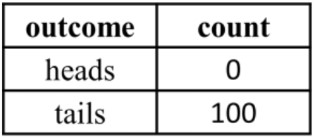# Theoretical vs. experimental probability

### Theoretical vs. experimental probability

In this lesson, we will learn:

• The difference between theoretical and experimental probability
• How to calculate the number of expected outcomes using theoretical probability and number of experimental trials
• How to write the experimental probability as a fraction based off the observed results in an experiment

Notes:

• Probability for simple events means we are just looking at one probability scenario at a time (i.e. one coin flip; a single six-sided die toss; one spinner)

• There are two types of probability:
• Theoretical probability is an educated guess on what you think should or will happen ("expected" probability; based on theory)
• Experimental probability is based on an experiment and what you saw happen already ("observed" probability; happened in reality)

• The probability we have seen so far in previous lessons is based on theoretical probability. We can use theoretical probability to find the number of expected outcomes.
• Before you do an experiment, you can predict how many times an outcome will happen (how many times it should theoretically happen)

$P$ (event) = $\frac{number\,outcomes\,wanted} {total\,number\,possible\,outcomes}$

• This is based on the number of trials you will do in the experiment. A trial is each run through of the experiment--1 trial will give 1 outcome (each coin flip, each dice toss, each spinner spin)

# expected outcomes = $P$ (event) × # trials

• The experimental probability is based off the observations made during the experiment and calculated once all trials are completed.

$P$ (experimental event) = $\frac{number\,outcomes\,observed} {total\,number\,trials}$

Ex. For an experiment, a coin is flipped 8 times. The results are shown in an observation table: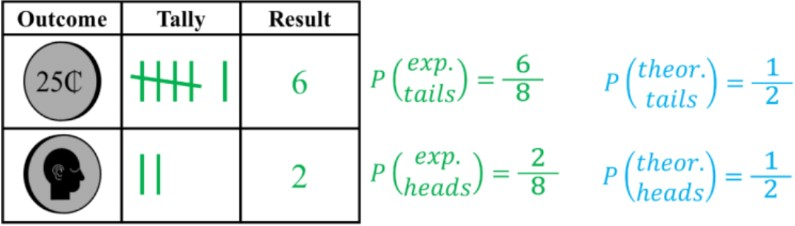• The experimental (exp.) probabilities do not match with the theoretical (theor.) probabilities.
• The more trials you do, the closer the results should get to the expected probabilities (i.e. doing 10 trials vs. 100 trials vs. 1000 trials, etc.)

#### Lessons

• Introduction
Introduction to Basic Probability for Simple Events:
a)
Theoretical probability and expected outcomes

b)
Finding experimental probability and comparing with experimental probability

c)
Giving experimental probability as a fraction

d)
Review on theoretical probability vs. experimental probability

• 1.
Theoretical probability and expectations
a)
How many times would you expect to land on heads if you flipped a coin 10 times?

b)
How many times would you expect to roll the number 2 if you toss a six-sided die 30 times?

c)
How many times would you expect to land on the letter A if you spin a four-part spinner 40 times?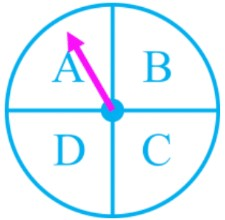d)
The experiment will consist of pulling 1 lollipop out of the bag at a time. Each lollipop is put back into the bag before the next pull.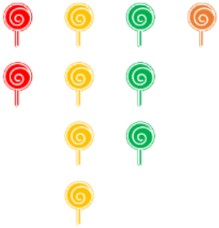1. How many times would you expect to pull a red lollipop if you tried 20 times?
2. How many times would you expect to pull a red lollipop if you tried 20 times?

e)
How many times would you expect to land on a number greater than 4 if you toss a six-sided die 9 times?

• 2.
Comparing experimental probability and theoretical probability (results in reality vs. expectation)
A student is preparing to conduct a probability experiment flipping a coin. They will record their results in a table: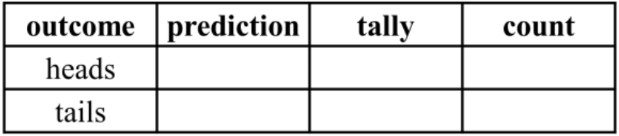a)
What (theoretical) probability fraction is expected for each outcome?

b)
Which of the following seems most likely to happen if the coin will be flipped 100 times?
1. 75 heads, 25 tails
2. 0 heads, 100 tails
3. 55 heads, 45 tails

c)
Write the experiment probability for P(heads) and P(tails) in the student's experiment with the completed results. Do the results match the expected probabilities? Explain.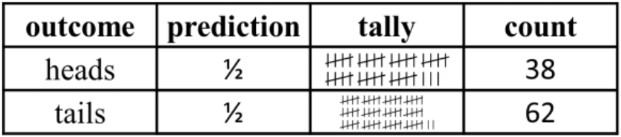d)
In this scenario, every time the coin was flipped, it landed on tails facing up. Normally this would be extremely rare (near impossible). What are some explanations for what happened?